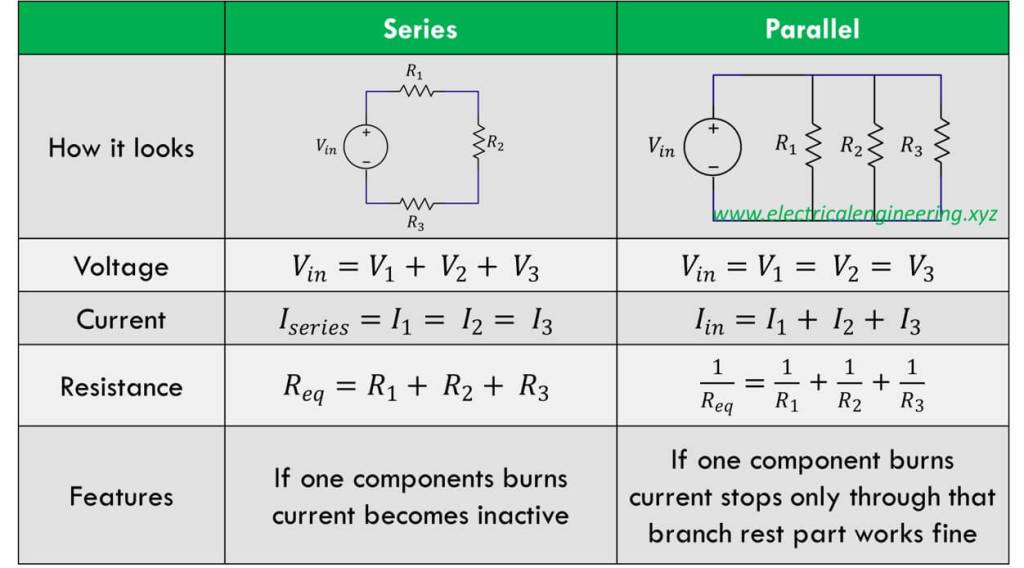# Parallel Circuits Study Guide

INTRODUCTION

When we compare series and parallel circuits, it is easy to analyze the circuit with only one battery and one load resistance. But what if the number of components are increased?

## MEANING OF PARALLEL CIRCUIT

• The parallel circuit is a very common and widely used circuit.
• A parallel circuit is the type of circuit that includes more than two separate paths.
• These paths allow the flow of current from the negative terminal of the component to the positive terminal of the component.

The laws of parallel circuits state that:

1. The total voltage of a parallel circuit is equal to the voltage all across the circuit.
2. The total current is the sum of the individual currents.
3. Total resistance is equal to the sum of individual resistance.

## DIAGRAM OF PARALLEL CIRCUIT

Parallel circuit diagram:Source

## EXAMPLES OF PARALLEL CIRCUITS

The parallel circuit examples are:

• Outlets in our home
• Washing machines
• Home wiring
• Wiring in toys
• Microwave
• Home appliances
• Industrial installation

## FORMULA FOR PARALLEL CIRCUIT

Parallel circuit formula:

1/Rt = 1/R1+1/R2+1/R3 … Rt = R (t)total.

## DIFFERENCE BETWEEN PARALLEL AND SERIES CIRCUITSource

# SUMMARY

• A circuit with more than one resistance is known as a parallel circuit.
• A parallel circuit is a type of circuit that includes more than two separate paths, allowing the flow of current from the negative terminal of the component to the positive terminal of the component.
• The difference between series and parallel circuits are:
• In series, there is one single node. While in parallel, it is more than two.
• The voltage is divided into series. While in parallel, it is the same all across.
• Resistors are simply connected to get equal resistance. Whereas in parallel, resistors need to get a specific formula.
• The parallel circuit formula: 1/Rt = 1/R1+1/R2+1/R3 … Rt = R (t)total.

## FAQs

Q. What are parallel circuits?

A parallel circuit is a type of circuit that includes more than two separate paths, allowing the flow of current from the negative terminal of the component to the positive terminal of the component.

Q. What is a parallel circuit example?

• Outlets in our home
• Washing machines
• Home wiring
• Wiring in toys
• Microwave
• Industrial installation

Q. What is the difference between series and parallel circuits?

In the parallel circuit, there is more than a single node and has maintained voltage all across the circuit. Whereas, in the series circuit, there is only a single node, along with the divided voltage in all the individual resistance.

Q. What are parallel circuits used for?

Parallel circuits are used for home appliances, industrial installation, home wiring, toy factories, etc.

We hope you enjoyed studying this lesson and learned something cool about Parallel circuits! Join our Discord community to get any questions you may have answered and to engage with other students just like you! We promise, it makes studying much more fun!😎

## REFERENCES

1. Series and Parallel Circuit: https://electricalacademia.com/basic-electrical/series-parallel-circuit-series-parallel-circuit-solved-problems/ Accessed 19th April 2022.
2. Series and Parallel Circuit:https://www.allaboutcircuits.com/textbook/direct-current/chpt-5/what-are-series-and-parallel-circuits/ Accessed 19th April 2022.
3. Parallel Circuit: https://www.linquip.com/blog/what-is-parallel-circuit/ Accessed 19th April 2022.
4. Parallel Circuit: https://www.britannica.com/technology/parallel-circuit Accessed 19th April 2022.
5. Differences Between Series and Parallel Circuits: https://www.electricalengineering.xyz/questions/top-5-differences-between-series-and-parallel-circuits/Accessed 19th April 2022.
]]>